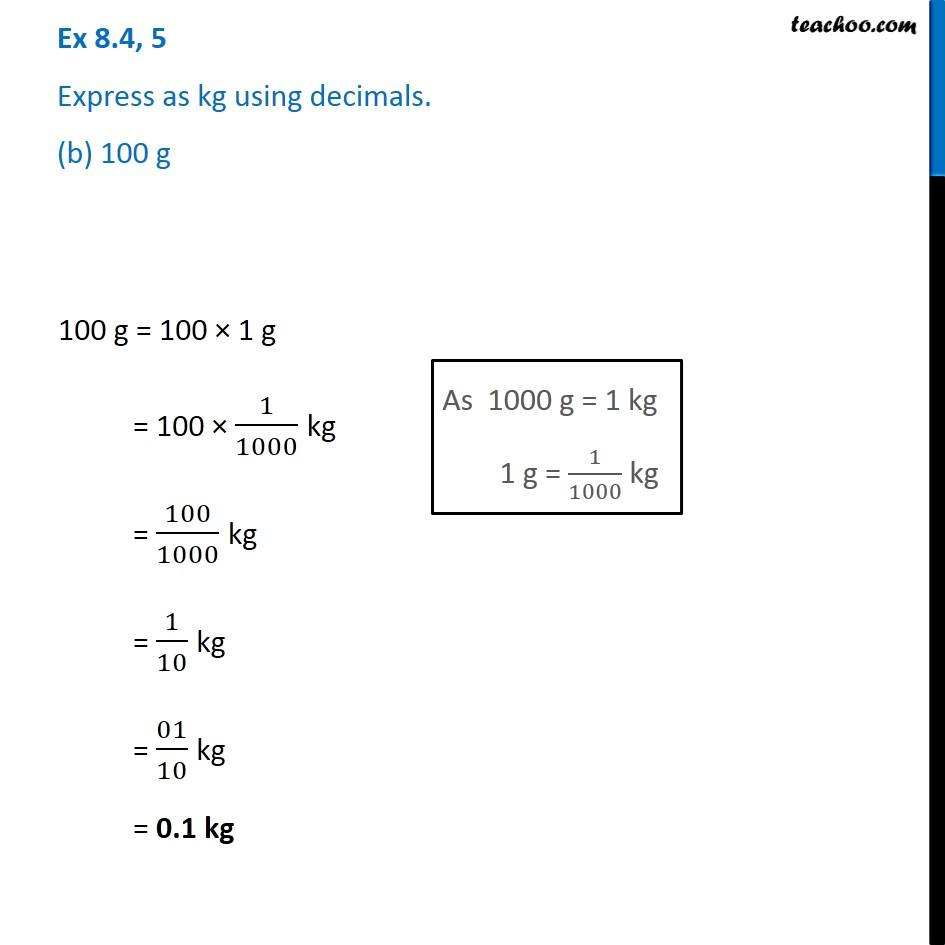1. Chapter 8 Class 6 Decimals
2. Serial order wise
3. Ex 8.4

Transcript

Ex 8.4, 5 Express as kg using decimals. (b) 100 g 100 g = 100 × 1 g = 100 × 1/1000 kg = 100/1000 kg = 1/10 kg = 01/10 kg = 0.1 kg